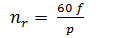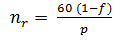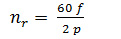##### Live Support##### OfflineElectrical Preliminary Test
1.
Which is the typical classification of electrical machines?
A.
Static and aeroderivative machines.
B.
Only rotating machines.
C.
Static and rotating machines.
2.
Which is the operating principle of the electric generator?
A.
To convert electrical power to mechanical power.
B.
To convert mechanical power to electrical power.
C.
To convert electrical power to thermal power.
3.
Which is the typical range of the electrical machines efficiency?
A.
40 - 45 %
B.
75 – 80 %
C.
95 – 99 %
4.
How is the speed of the rotor calculated? (f: frequency; p: pole pairs; nr: speed of the rotor)
A.B.C.5.
What does AVR mean?
A.
Automatic voltage regulator.
B.
Automatic speed regulator.
C.
Automatic frequency regulator.
6.
When may a current peak occur?
A.
During the motor start up.
B.
During the motor switch off.
C.
During the stationary regime.
7.
What are the main parts of the stator?
A.
Only a magnetic core.
B.
Magnetic core and brushes.
C.
Magnetic core and windings.
8.
What is the formula for Reactive Power?
A.
Q = V I
B.
Q = V I cos φ
C.
Q = V I sin φ
9.
What is the meaning of P.I. and I.R. for insulation testing?
A.
Protection index and insulation resistance.
B.
Polarization index and insulation resistance.
C.
Piping and instrumentation and insulation resistance.
10.
What is the main function of the jacking oil system?
A.
To lift the rotor and allowing the revolution to begin.
B.
To cool the motor.
C.
To pressurize the entire system.
11.
What are the main components of VFD?
A.
Rectifier only.
B.
Rectifier, filter and inverter.
C.
Rectifier and inverter.
12.
What is the power factor?
A.
Angle between voltage and current.
B.
Angle between voltage and frequency.
C.
Cos of angle between voltage and current.